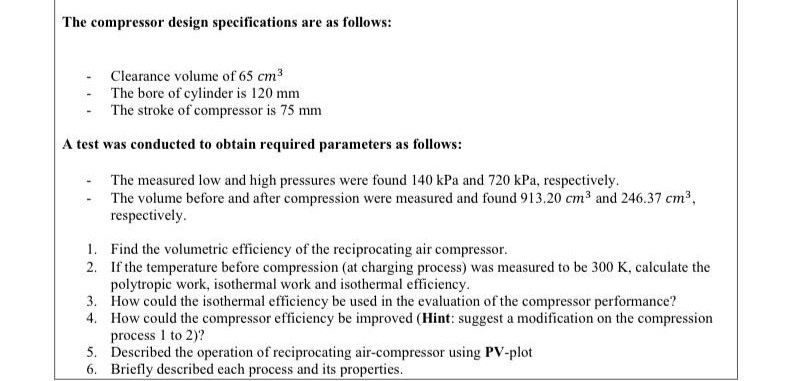# The compressor design specifications are as follows: Clearance volume of 65 cm3 The bore of cylinder is 120 mm - The stroke of compressor is 75 mm A test was conducted to obtain required parameters as follows: The measured low and high pressures were found 140 kPa and 720 kPa, respectively. - The volume before and after compression were measured and found 913.20 cm³ and 246.37 cm3, respectively. 1. Find the volumetric efficiency of the reciprocating air compressor. 2. If the temperature before compression (at charging process) was measured to be 300 K, calculate the polytropic work, isothermal work and isothermal efficiency. 3. How could the isothermal efficiency be used in the evaluation of the compressor performance? 4. How could the compressor efficiency be improved (Hint: suggest a modification on the compression process I to 2)? 5. Described the operation of reciprocating air-compressor using PV-plot 6. Briefly described each process and its properties.

Question

Can you solve it?help_outlineImage TranscriptioncloseThe compressor design specifications are as follows: Clearance volume of 65 cm3 The bore of cylinder is 120 mm - The stroke of compressor is 75 mm A test was conducted to obtain required parameters as follows: The measured low and high pressures were found 140 kPa and 720 kPa, respectively. - The volume before and after compression were measured and found 913.20 cm³ and 246.37 cm3, respectively. 1. Find the volumetric efficiency of the reciprocating air compressor. 2. If the temperature before compression (at charging process) was measured to be 300 K, calculate the polytropic work, isothermal work and isothermal efficiency. 3. How could the isothermal efficiency be used in the evaluation of the compressor performance? 4. How could the compressor efficiency be improved (Hint: suggest a modification on the compression process I to 2)? 5. Described the operation of reciprocating air-compressor using PV-plot 6. Briefly described each process and its properties. fullscreen

### Want to see this answer and more?

Experts are waiting 24/7 to provide step-by-step solutions in as fast as 30 minutes!*

*Response times vary by subject and question complexity. Median response time is 34 minutes and may be longer for new subjects.
Tagged in
EngineeringMechanical Engineering

### Powerplant Engineering